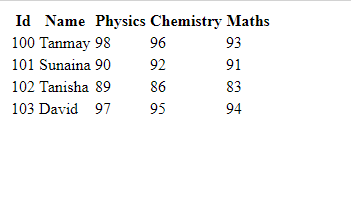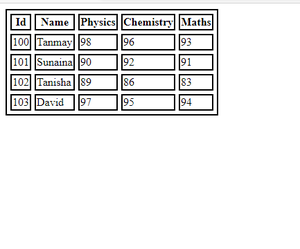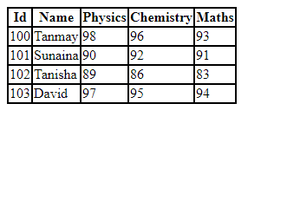Open in App
Not now

# How to set the collapsing borders model for a table ?

• Last Updated : 01 Apr, 2021

CSS describes the way HTML elements should be displayed. There are various CSS properties that can add magic to the basic HTML document.

HTML tables allow us to arrange our data using rows and columns. The <table> tag defines an HTML table. Each table row is defined with a <tr> tag. Each table header is defined with a <th> tag. Each table data/cell is defined with a <td> tag. Let us see how we can construct a table that can represent the marks of four students in respective subjects and set the collapsed borders for it as shown below.

Example 1: This example describes the basic example of HTML table.

## HTML

 ```<``html``>`` ` `<``head``>``    ``<``title``>css collapse borders```` ` `<``body``>``    ``<``table``>``        ``<``tr``>``            ``<``th``>Id``            ``<``th``>Name``            ``<``th``>Physics``            ``<``th``>Chemistry``            ``<``th``>Maths``        ````        ``<``tr``>``            ``<``td``>100``            ``<``td``>Tanmay``            ``<``td``>98``            ``<``td``>96``            ``<``td``>93``        ````        ``<``tr``>``            ``<``td``>101``            ``<``td``>Sunaina``            ``<``td``>90``            ``<``td``>92``            ``<``td``>91``        ````        ``<``tr``>``            ``<``td``>102``            ``<``td``>Tanisha``            ``<``td``>89``            ``<``td``>86``            ``<``td``>83``        ````        ``<``tr``>``            ``<``td``>103``            ``<``td``>David``            ``<``td``>97``            ``<``td``>95``            ``<``td``>94``        ````    `````` ` ``

Output:HTML Table

Example 2: In this example, we will add some borders to it using the CSS border property as shown in the below example.

## HTML

 ```<``html``>`` ` `<``head``>``    ``<``title``>CSS border collapse``    ``<``style``>``        ``table,``        ``tr,``        ``th,``        ``td {``            ``border: 2px solid black;``            ``border-spacing: 5px;``        ``}``    `````` ` `<``body``>``    ``<``table``>``        ``<``tr``>``            ``<``th``>Id``            ``<``th``>Name``            ``<``th``>Physics``            ``<``th``>Chemistry``            ``<``th``>Maths``        ````        ``<``tr``>``            ``<``td``>100``            ``<``td``>Tanmay``            ``<``td``>98``            ``<``td``>96``            ``<``td``>93``        ````        ``<``tr``>``            ``<``td``>101``            ``<``td``>Sunaina``            ``<``td``>90``            ``<``td``>92``            ``<``td``>91``        ````        ``<``tr``>``            ``<``td``>102``            ``<``td``>Tanisha``            ``<``td``>89``            ``<``td``>86``            ``<``td``>83``        ````        ``<``tr``>``            ``<``td``>103``            ``<``td``>David``            ``<``td``>97``            ``<``td``>95``            ``<``td``>94``        ````    `````` ` ``

Output:HTML table with borders

On adding the borders to our table, we get the output as shown above. But this may not be the required output, So let us try to collapse these borders to get a more unified output using the border-collapse property and setting its value to collapse as shown in the next example.

Example 3:

## HTML

 ```<``html``>`` ` `<``head``>``    ``<``title``>CSS border collapse``    ``<``style``>``        ``table,``        ``tr,``        ``th,``        ``td {``            ``border: 2px solid black;``            ``border-collapse: collapse;``            ``border-spacing: 5px;``        ``}``    `````` ` `<``body``>``    ``<``table``>``        ``<``tr``>``            ``<``th``>Id``            ``<``th``>Name``            ``<``th``>Physics``            ``<``th``>Chemistry``            ``<``th``>Maths``        ````        ``<``tr``>``            ``<``td``>100``            ``<``td``>Tanmay``            ``<``td``>98``            ``<``td``>96``            ``<``td``>93``        ````        ``<``tr``>``            ``<``td``>101``            ``<``td``>Sunaina``            ``<``td``>90``            ``<``td``>92``            ``<``td``>91``        ````        ``<``tr``>``            ``<``td``>102``            ``<``td``>Tanisha``            ``<``td``>89``            ``<``td``>86``            ``<``td``>83``        ````        ``<``tr``>``            ``<``td``>103``            ``<``td``>David``            ``<``td``>97``            ``<``td``>95``            ``<``td``>94``        ````    `````` ` ``

Output:Table with collapsed borders

This is our required output, So finally we have learned how to set the collapsed borders for a table. The point to remember is that the border-spacing property will have no effect on the border-collapse property when set to collapse value.

My Personal Notes arrow_drop_up